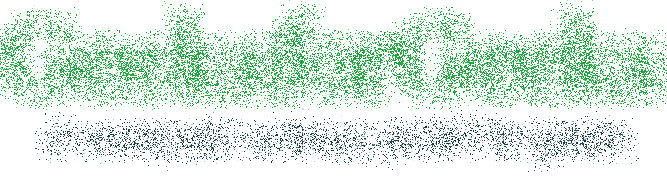Related Articles

# Wand spread() function – Python

• Last Updated : 18 Aug, 2021

The spread() function is an inbuilt function in the Python Wand ImageMagick library which is used to replace each pixel with the statistic results from neighboring pixel values. The width & height defines the size, or aperture, of the neighboring pixels.

Syntax:

`spread(radius, method)`

Parameters: This function accepts two parameters as mentioned above and defined below:

• radius: This parameter stores the radius of the pixel can be displaced from source.
• method: This parameter stores the available color methods. Some of the available methods are ‘undefined’, ‘average’, ‘average9’, ‘average16’, ‘background’, ‘bilinear’, ‘blend’, ‘catrom’, ‘integer’, ‘mesh’, ‘nearest’, ‘spline’.

Return Value: This function returns the Wand ImageMagick object.

Original Image:Example 1:

## Python3

 `# Import library from Image``from` `wand.image ``import` `Image` `# Import the image``with Image(filename ``=``'../geeksforgeeks.png'``) as image:``    ``# Clone the image in order to process``    ``with image.clone() as spread:``        ``# Invoke spread function with radius 15 and method as 'nearest'``        ``spread.spread(``15``, ``'nearest'``)``        ``# Save the image``        ``spread.save(filename ``=``'spread1.jpg'``)`

Output:Example 2:

## Python3

 `# Import libraries from the wand ``from` `wand.image ``import` `Image``from` `wand.drawing ``import` `Drawing``from` `wand.color ``import` `Color` `with Drawing() as draw:``    ``# Set Stroke color the circle to black``    ``draw.stroke_color ``=` `Color(``'black'``)``    ``# Set Width of the circle to 2``    ``draw.stroke_width ``=` `1``    ``# Set the fill color to 'White (# FFFFFF)'``    ``draw.fill_color ``=` `Color(``'white'``)` `    ``# Invoke Circle function with center at 50, 50 and radius 25``    ``draw.circle((``200``, ``200``), ``# Center point``                ``(``100``, ``100``)) ``# Perimeter point``    ``# Set the font style``    ``draw.font ``=` `'../Helvetica.ttf'``    ``# Set the font size``    ``draw.font_size ``=` `30``    ` `    ``with Image(width ``=` `400``, height ``=` `400``, background ``=` `Color(``'# 45ff33'``)) as pic:``        ``# Set the text and its location``        ``draw.text(``int``(pic.width ``/` `3``), ``int``(pic.height ``/` `2``), ``'GeeksForGeeks !'``)``        ``# Draw the picture``        ``draw(pic)``        ``# Invoke spread function with radius 10 and method as 'blend'``        ``pic.spread(``10``, ``'blend'``)``        ``# Save the image``        ``pic.save(filename ``=``'spread2.jpg'``)`

Output:Attention geek! Strengthen your foundations with the Python Programming Foundation Course and learn the basics.

To begin with, your interview preparations Enhance your Data Structures concepts with the Python DS Course. And to begin with your Machine Learning Journey, join the Machine Learning – Basic Level Course

My Personal Notes arrow_drop_up